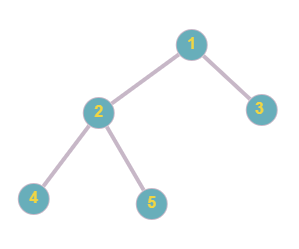# Postorder Traversal

Posted: 22 Jul, 2021
Difficulty: Easy

## PROBLEM STATEMENT

#### You are given the root node of a binary tree consisting of ‘N’ nodes. Your task is to return its postorder traversal. The postorder traversal of a binary tree is defined as a process of traversing each node in the following manner-:

``````1- Traverse all nodes in the left subtree of the root node.
2- Traverse all the nodes in the right subtree of the root node.
3- Visit the root node.
``````
##### For Example:
``````For the given tree below,
Postorder traversal for the given tree will be [4, 5, 2, 3, 1]. Hence, the answer is [4, 5, 2, 3, 1].
``````##### Example:
``````Elements are in the level order form. The input consists of values of nodes separated by a single space in a single line. In case a node is null, we take -1 in its place.

For example, the input for the tree depicted in the below image would be :
````````````1
2 3
4 -1 5 6
-1 7 -1 -1 -1 -1
-1 -1

Explanation :
Level 1 :
The root node of the tree is 1

Level 2 :
Left child of 1 = 2
Right child of 1 = 3

Level 3 :
Left child of 2 = 4
Right child of 2 = null (-1)
Left child of 3 = 5
Right child of 3 = 6

Level 4 :
Left child of 4 = null (-1)
Right child of 4 = 7
Left child of 5 = null (-1)
Right child of 5 = null (-1)
Left child of 6 = null (-1)
Right child of 6 = null (-1)

Level 5 :
Left child of 7 = null (-1)
Right child of 7 = null (-1)

The first not-null node (of the previous level) is treated as the parent of the first two nodes of the current level.

The second not-null node (of the previous level) is treated as the parent node for the next two nodes of the current level and so on.

The input ends when all nodes at the last level are null (-1).
``````
##### Note :
``````The above format was just to provide clarity on how the input is formed for a given tree.

The sequence will be put together in a single line separated by a single space. Hence, for the above-depicted tree, the input will be given as:

1 2 3 4 -1 5 6 -1 7 -1 -1 -1 -1 -1 -1
``````
##### Input Format:
``````The first line of the input contains a single integer, 'T,’ denoting the number of test cases.

The first line of each test case contains the elements of the tree in the level order form separated by a single space. If any node does not have a left or right child, take -1 in its place. Refer to the example for further clarification.
``````
##### Output Format:
``````For each test case, print a single line of space-separated integers denoting the post-order traversal of the given binary tree.

Print the output of each test case separate line.
``````
##### Note:
``````You do not need to print anything. It has already been taken care of. Just implement the function.
``````
##### Constraints:
``````1 <= T <= 10
1 <= N <= 10^4
0 <= nodeVal <= 10^9

Time Limit: 1 sec
``````Approach 1

In this approach, we will create a recursive function postorder(node, ans) that will traverse all the nodes and store the postorder traversal in an array ans. We will first recursively call this function for its left and right subtree to traverse the nodes in the left and right subtree, and then insert the node’s value into ans array for each node.

Algorithm:

• Defining the postorder(node, ans)
• If the node is an empty node, we will return from the function.
• Now we will traverse to the left subtree.
• Create a recursive call postorder(left child of the node, ans).
• Now we will traverse to the right subtree.
• Create a recursive call postorder(right child of the node, ans).
• Insert node value into the ans array
• We will define an array ans to store the postorder traversal of the given tree.
• We will call postorder(root, ans).
• Return the array ans.